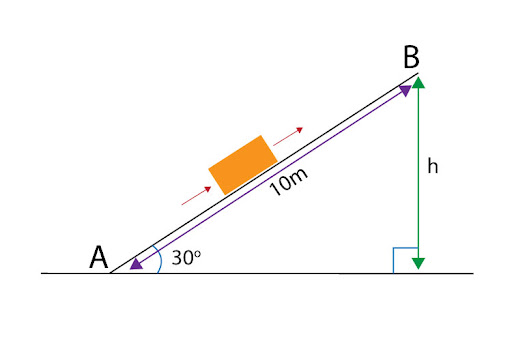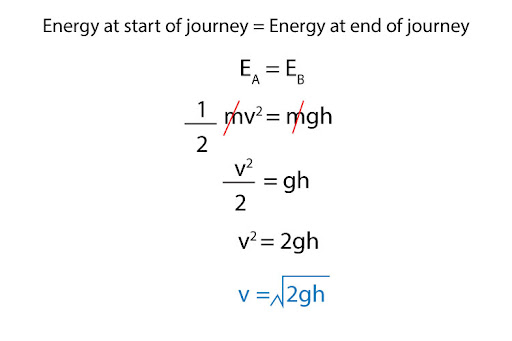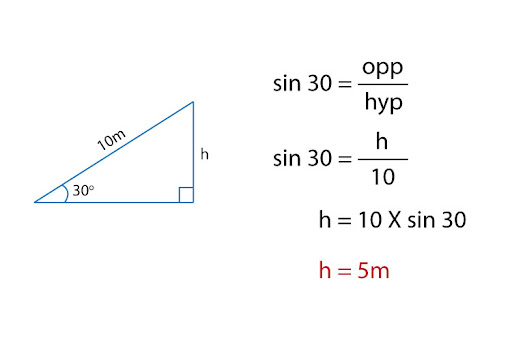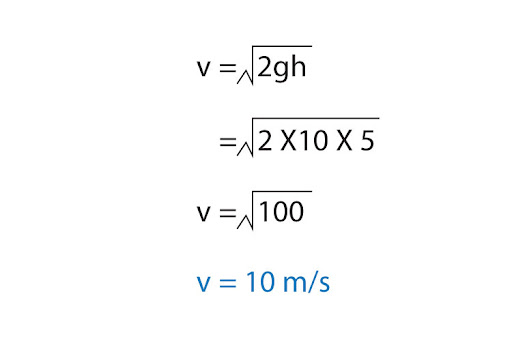# "A rollercoaster carriage wants to go up a slope of length 10m at an angle of 30 degrees above the horizontal, at what speed must the carriage be traveling at the bottom of the slope in order for it to reach the top? (Negligable Drag)"

A: This is what we call a journey-based question, wherein an object is travelling from one starting point to an ending point. Here, a rollercoaster carriage is moving up from the bottom of the slope, and stopping at the top of the slope.To solve such journey-based questions, we’ll use the Principle of Conservation of energy to our advantage: since energy cannot be created nor can it be destroyed, it can only transform from one form to another, such that the total energy remains constant.

At the bottom of the slope, the carriage only has kinetic energy because it is in motion. At the top of the slope, it has only gravitational potential energy. Note that due to negligible drag, there is no work done against frictional or resistive forces. Since total energy is constant throughout, energy at the start (at A) equals energy at the end (at B):Now, we can use trigonometry to find h:And then, it is simply a matter of inserting all the relevant values into your equation!